# SuiteCRM Calculation Field

Create business logic for field calculations without needing to write custom code. You can select calculated field and create the formula that is automatically updated based on calculation you provide.

## Grow your business using Calculated Fields to automate manual calculations & lessen the code for complex calculations

### Key Features

• You can give a field in your formula for dynamic calculations
• Available Functions : Add, Subtract, Multiply, Divide, String Length, Concatenation, Log(Logarithm), Ln(Natural Log), Absolute, Average, Power, Date Difference, Percentage, Mod(Modulo), Minimum, Negate, Floor, Ceil ,Add hours,Add day,Add week,Add month,Add year,Sub hours,Sub days,Sub week,Sub month,Sub year,Diff days,Diff hour,Diff minute,Diff month,Week Diff,Diff year
• A rich conditional support we provide: you can make a big calculation here, a nested formula
• You can insert manual value or Field or both together to create formula. For e.g. add(field1,200,sub(100,field2))
• You can use calculated field also to make further formula

### Screen Shots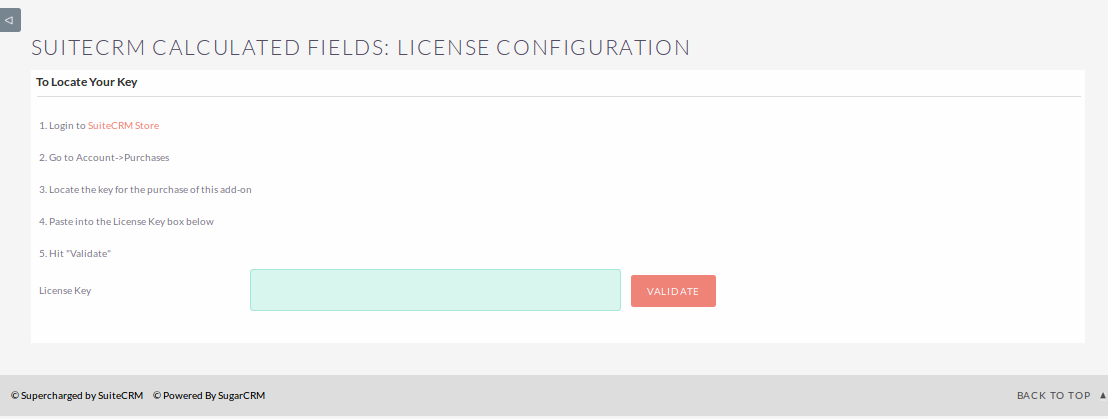Admin -> Repair -> Do 'Quick Repair & Rebuild'

• Studio -> 'Module' -> Add Fields -> Select 'Data Type' : Calculated Field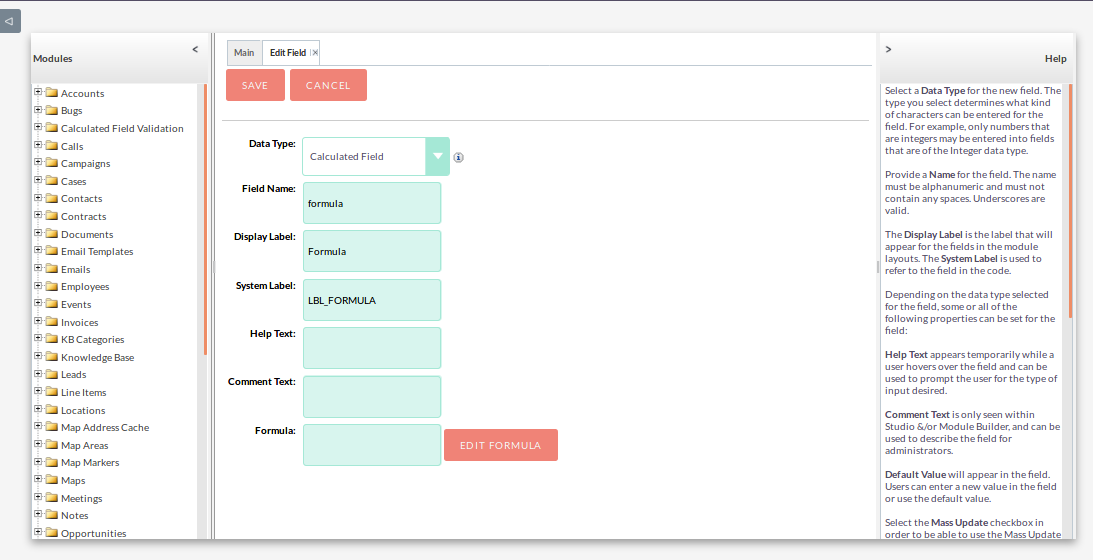• Click ‘Edit Formula’ to write your formula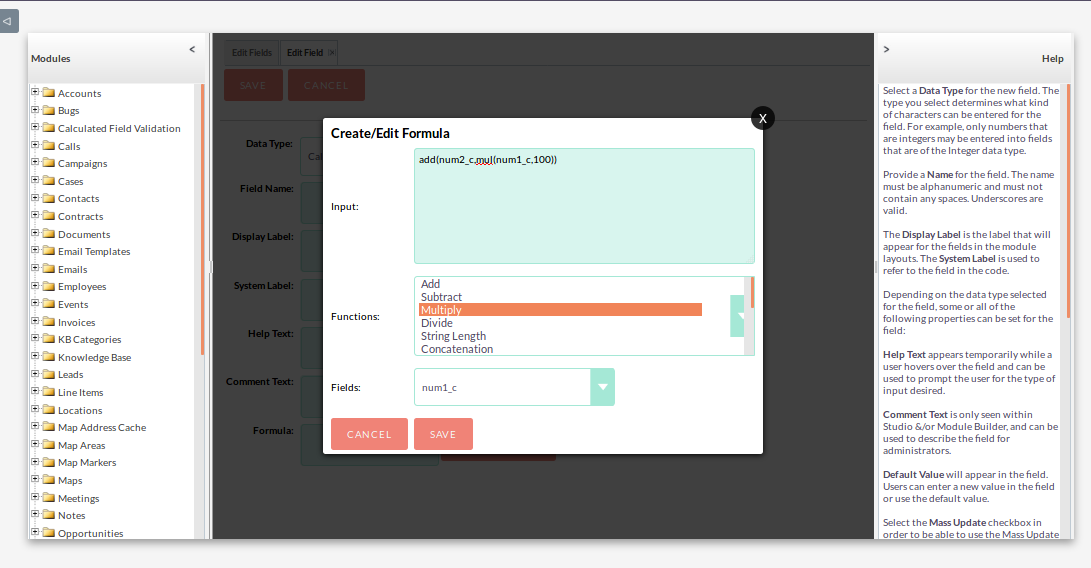Result of the above formula• Calculate Percentage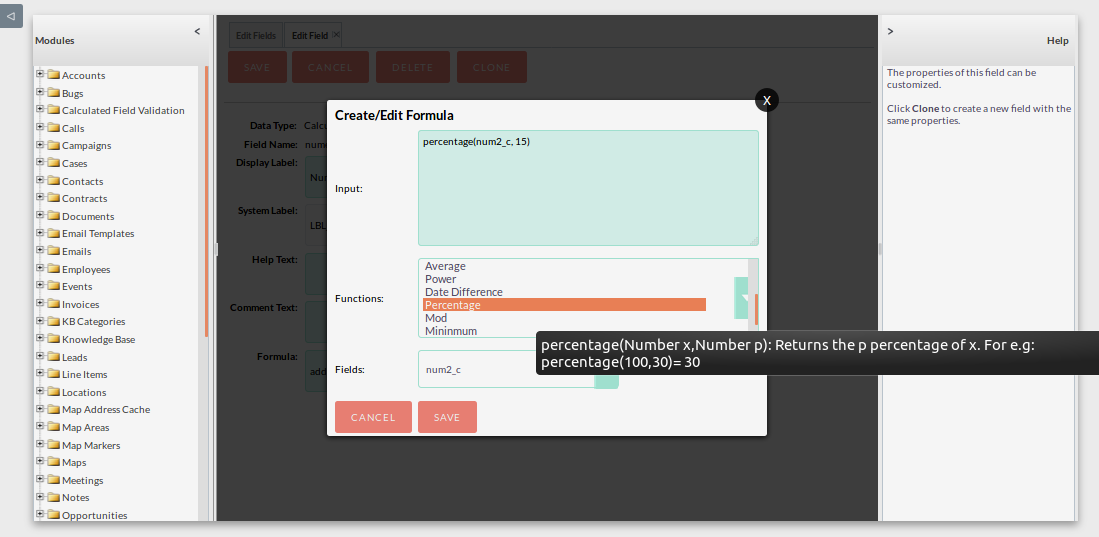Result of Percentage Formula• Calculate Date Difference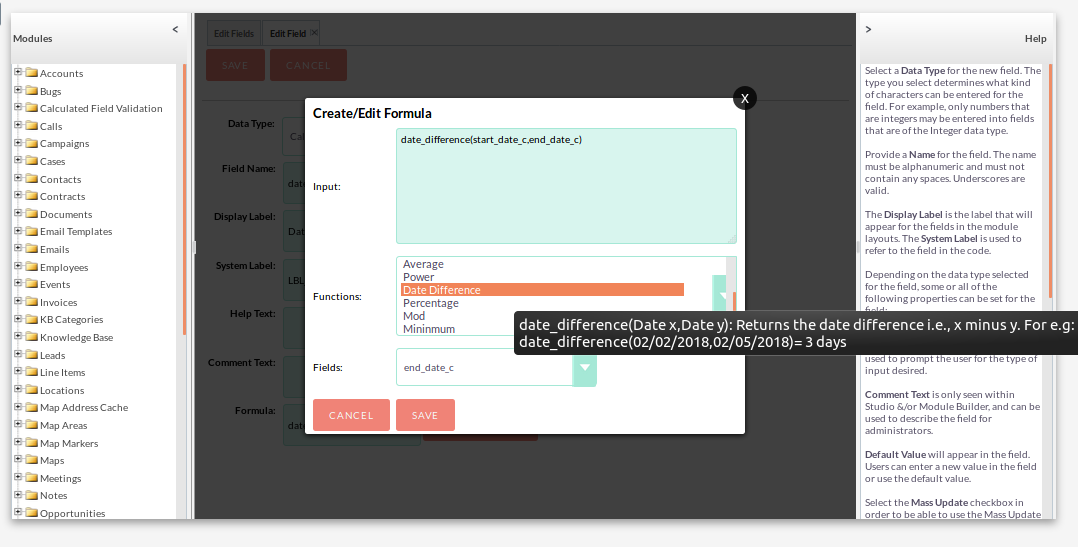Result of Date Difference• Concatenation Example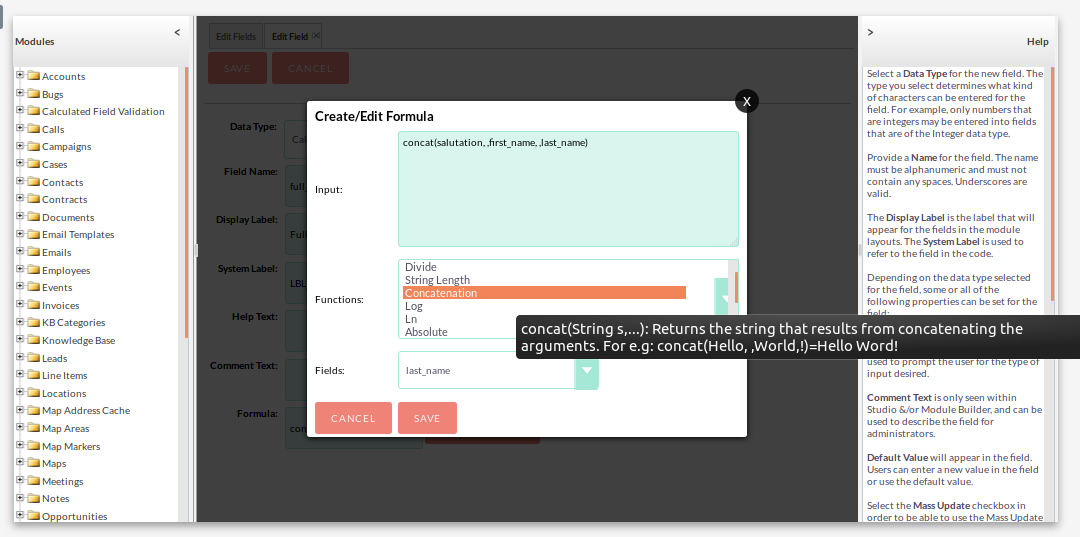Result of Concatenation• Calculate Subtract Month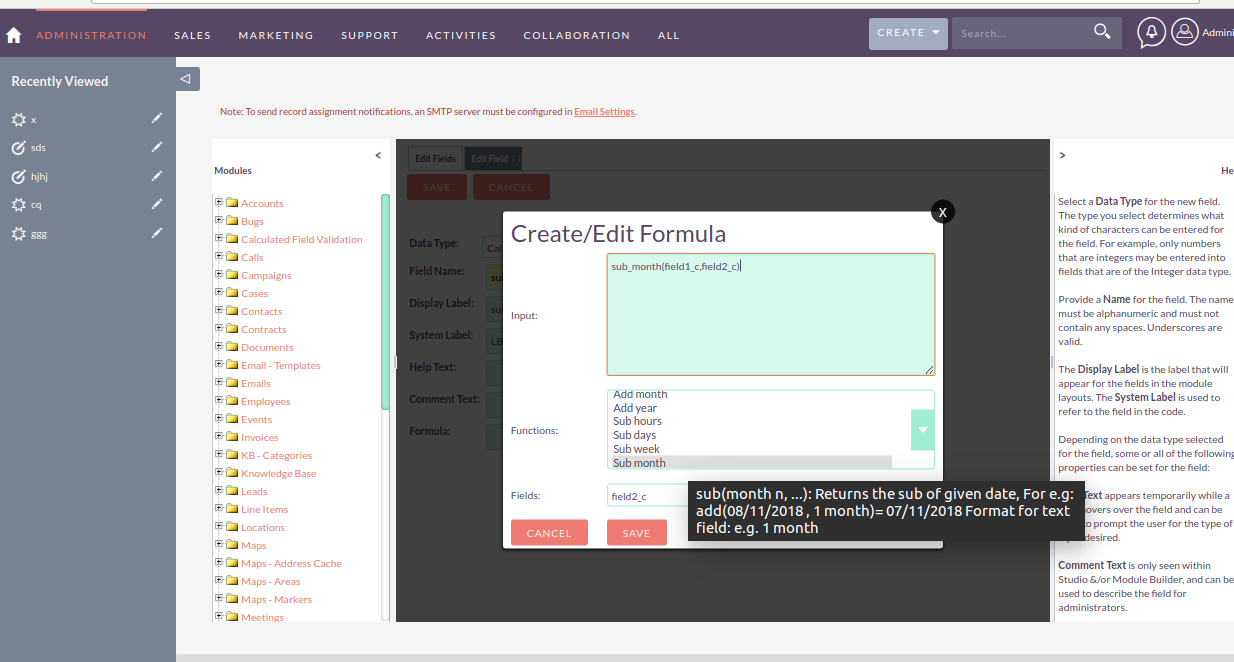Result of Subtract Month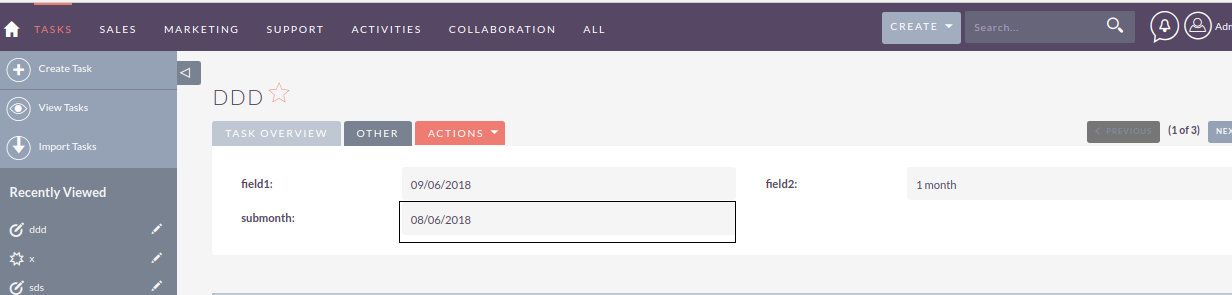• Calculate Difference Minute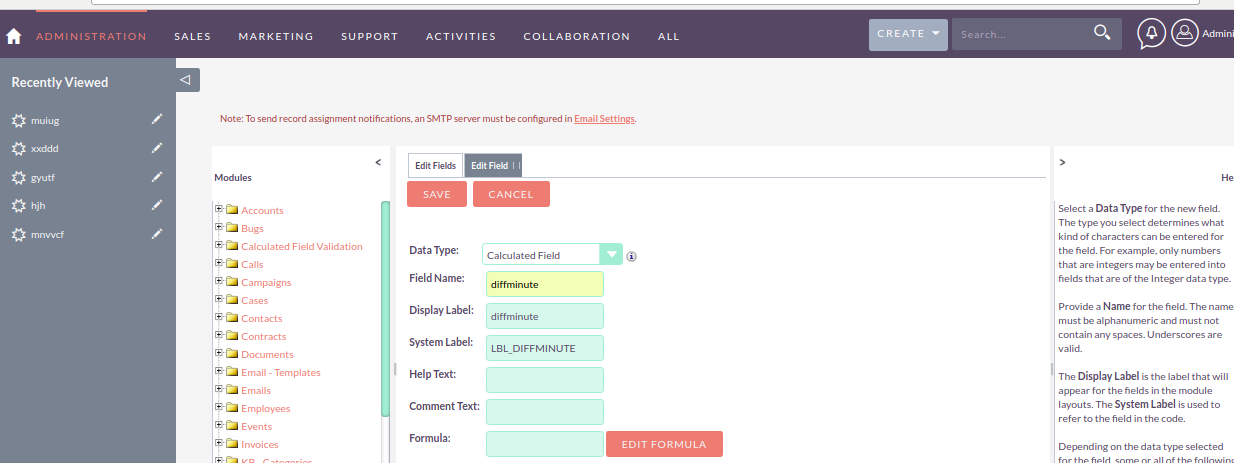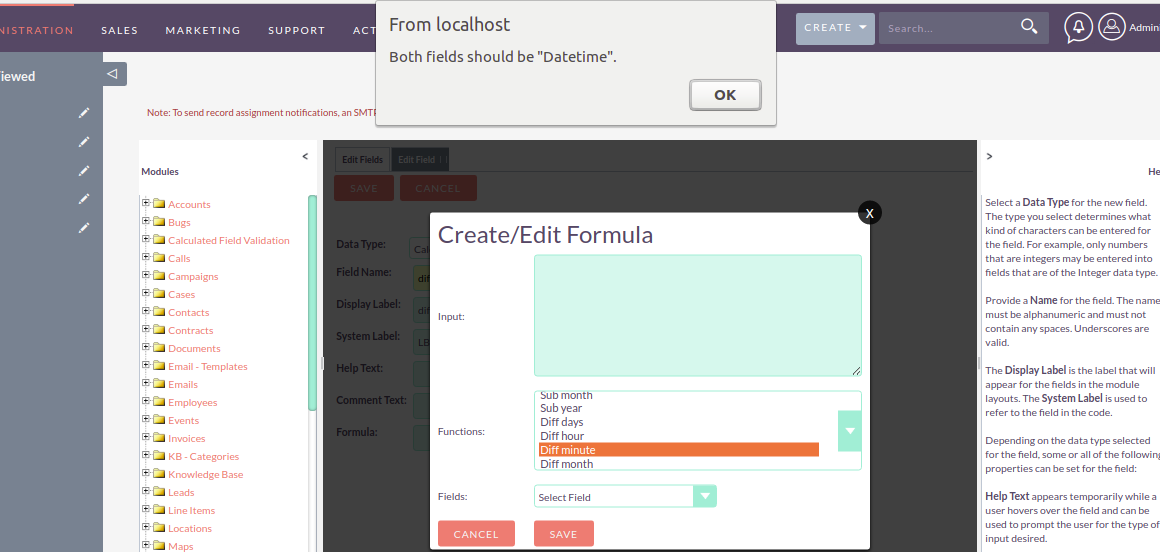Result of Minute Difference* Free 30 day trial